Case Based Questions (MCQ)

Chapter 2 Class 10 Polynomials
Serial order wise

## For a linear polynomial kx + c, k ≠ 0, the graph  of y = kx + c is a straight line which intersects  the X-axis at exactly one point, namely, ((-c)/k,0), Therefore, the linear polynomial kx + c, k ≠ 0, has  exactly one zero, namely, the X-coordinate of the  point where the graph of y = kx + c intersects the  X-axis.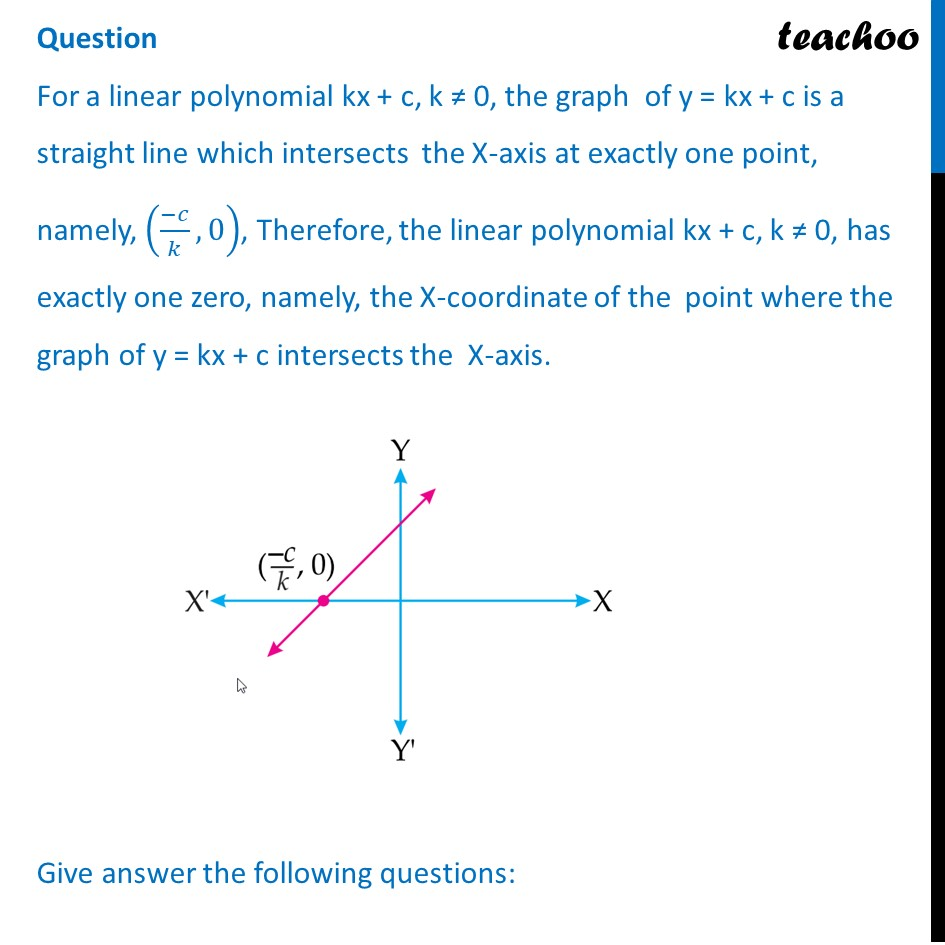## (d) − 2/3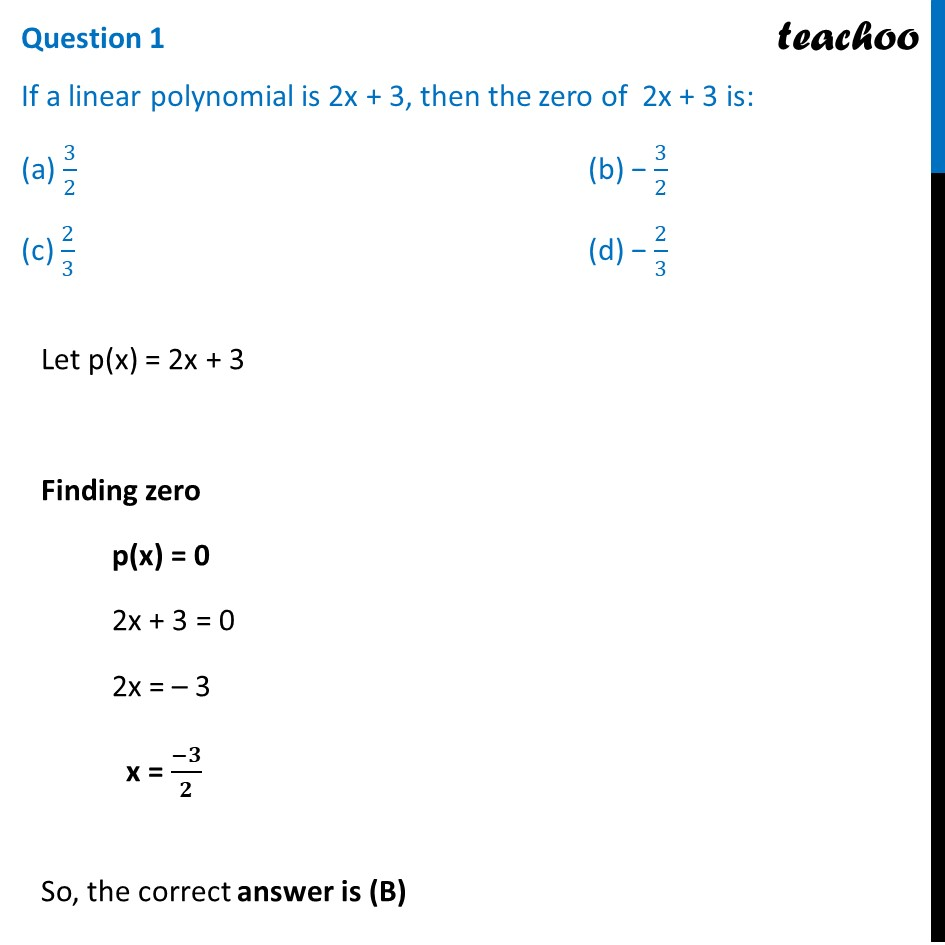## (d) 0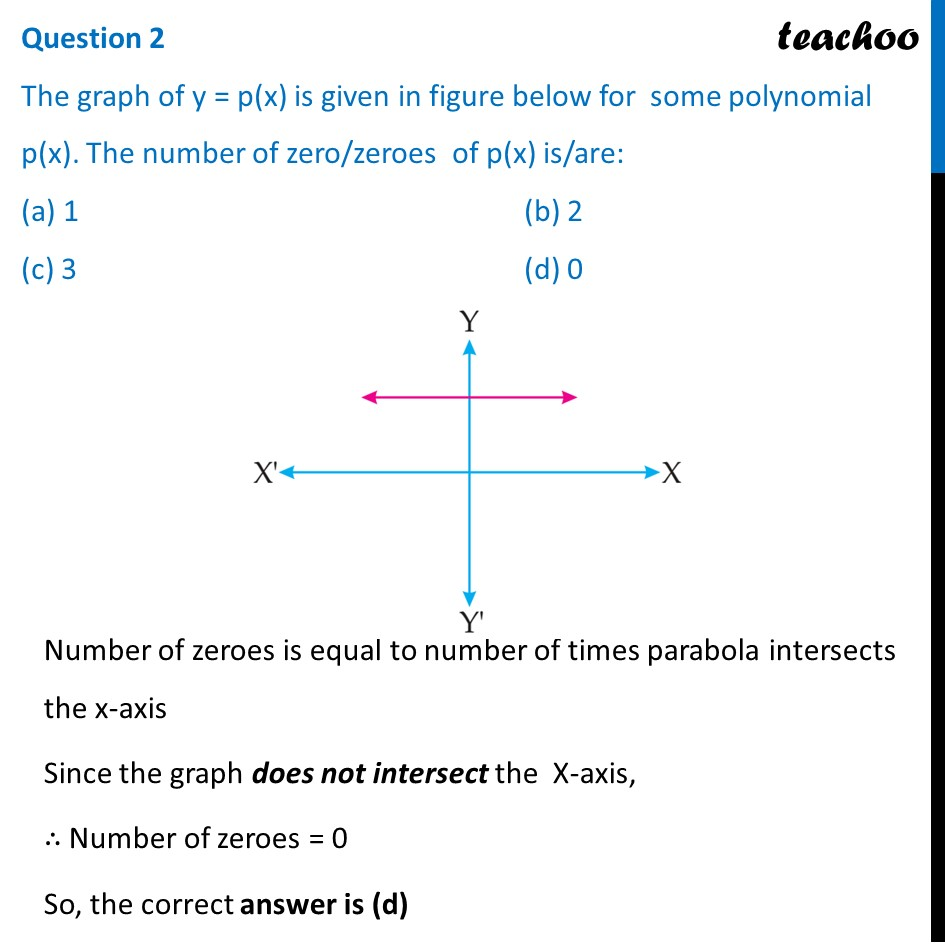## (d) 3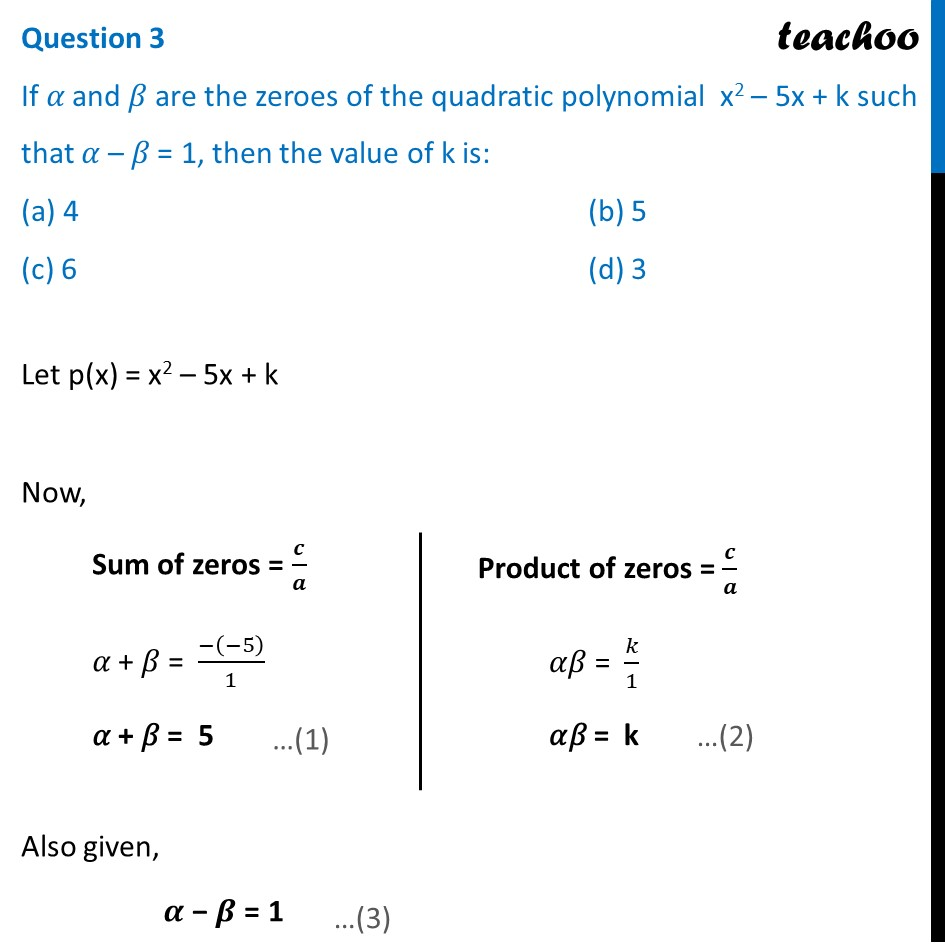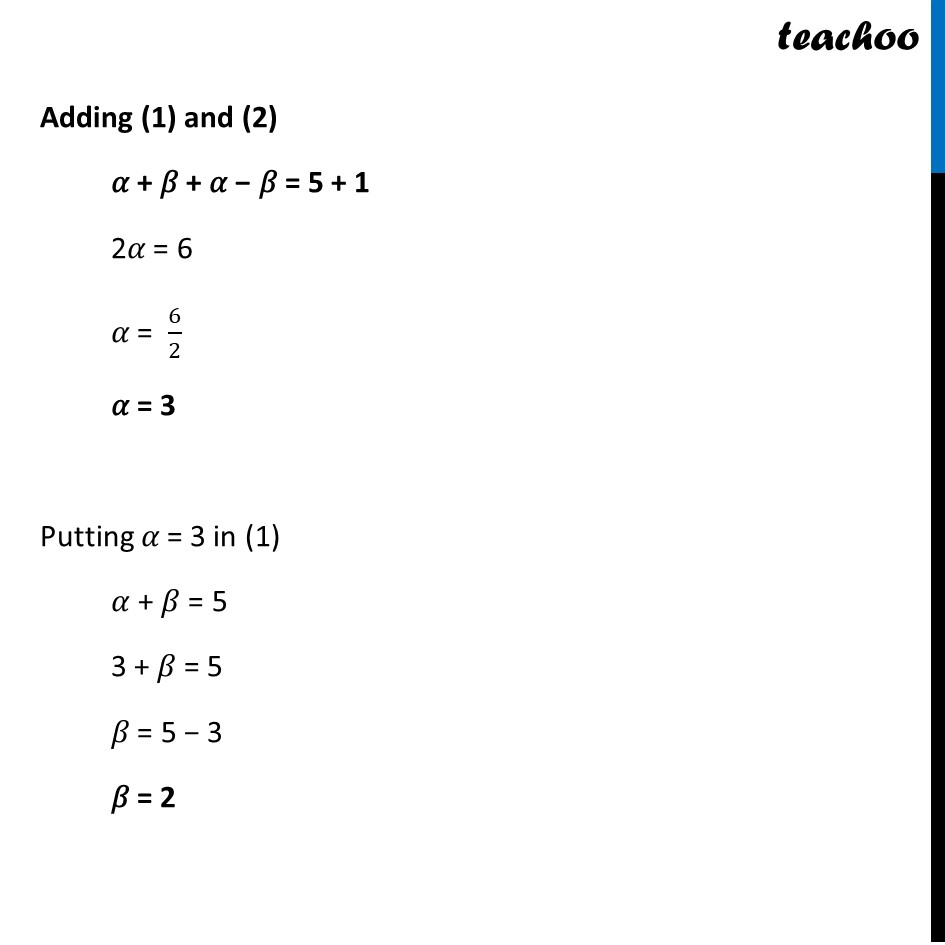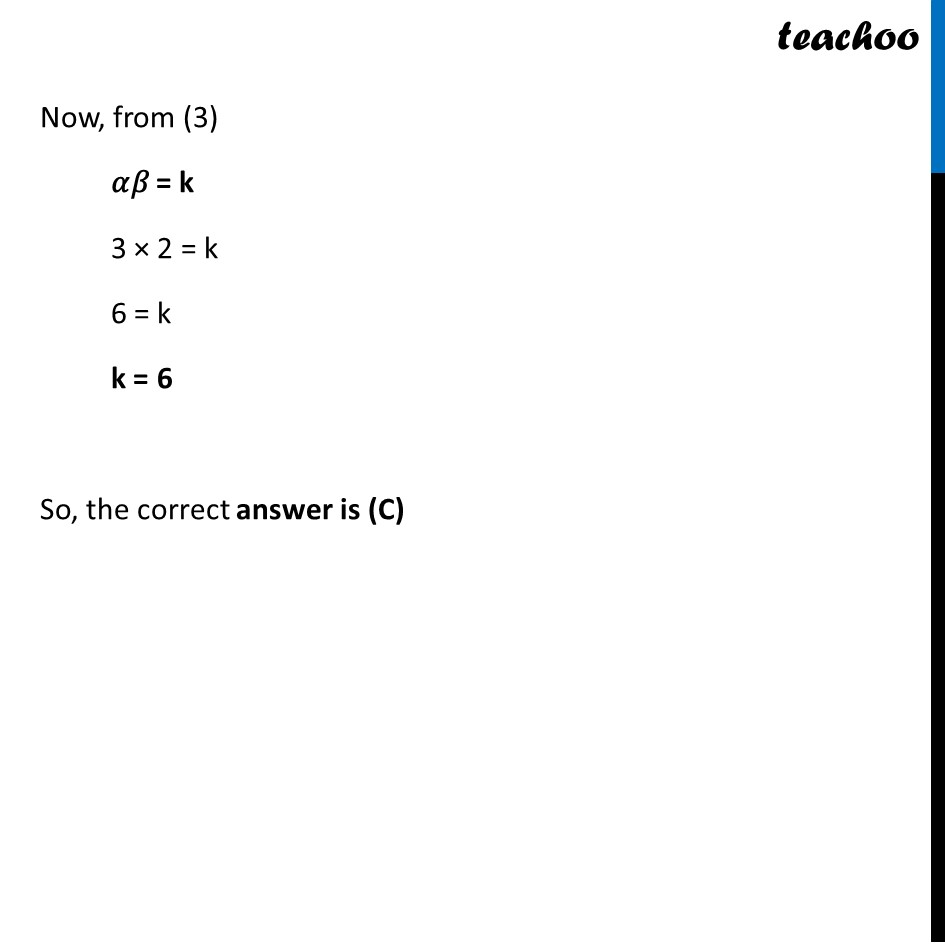## (d) − 5/4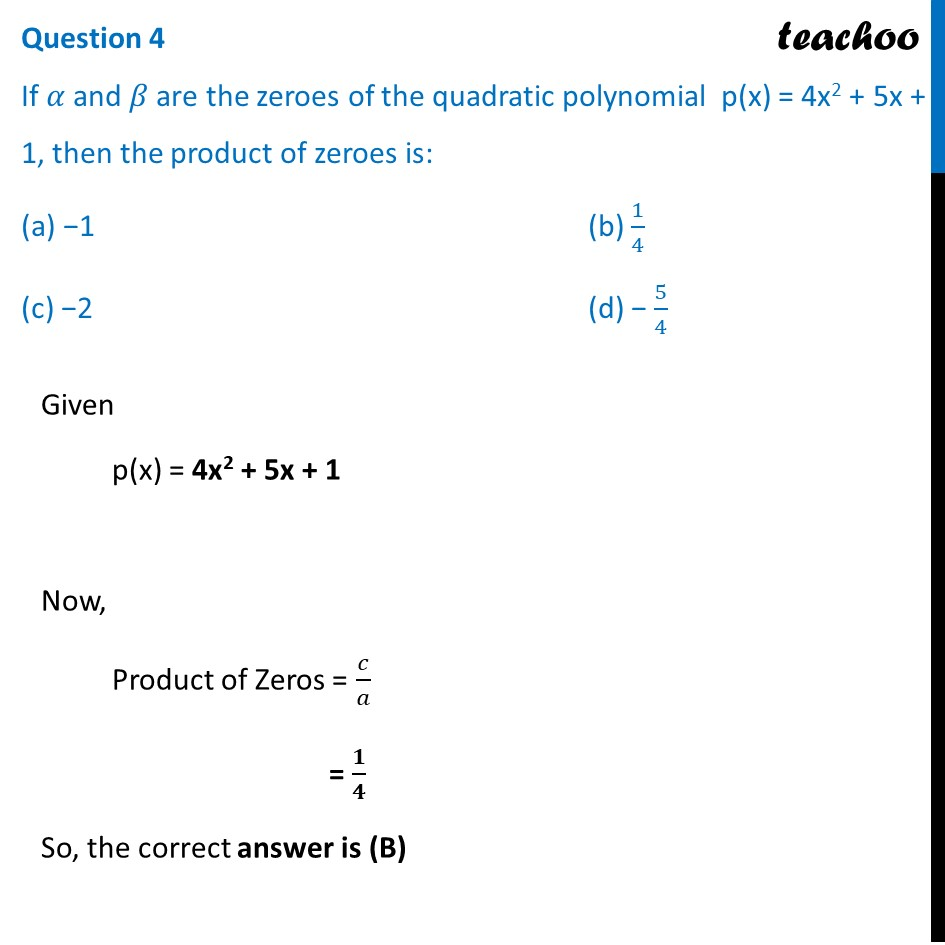## (d) − 2/3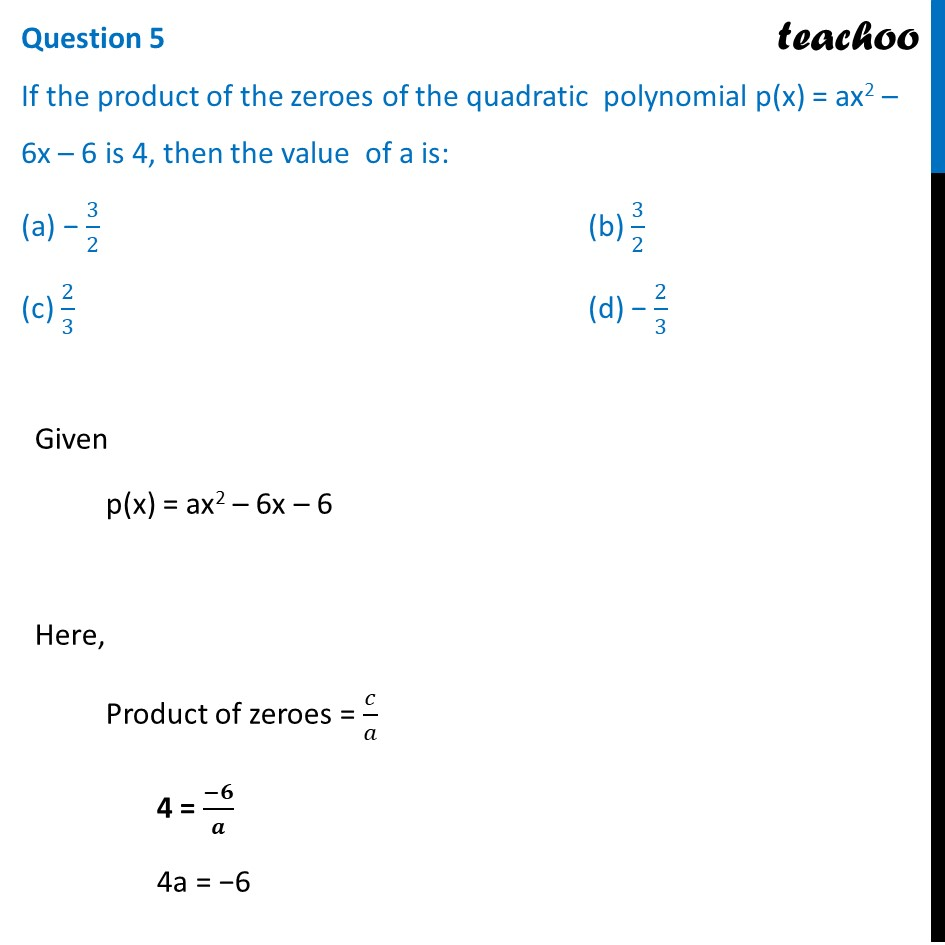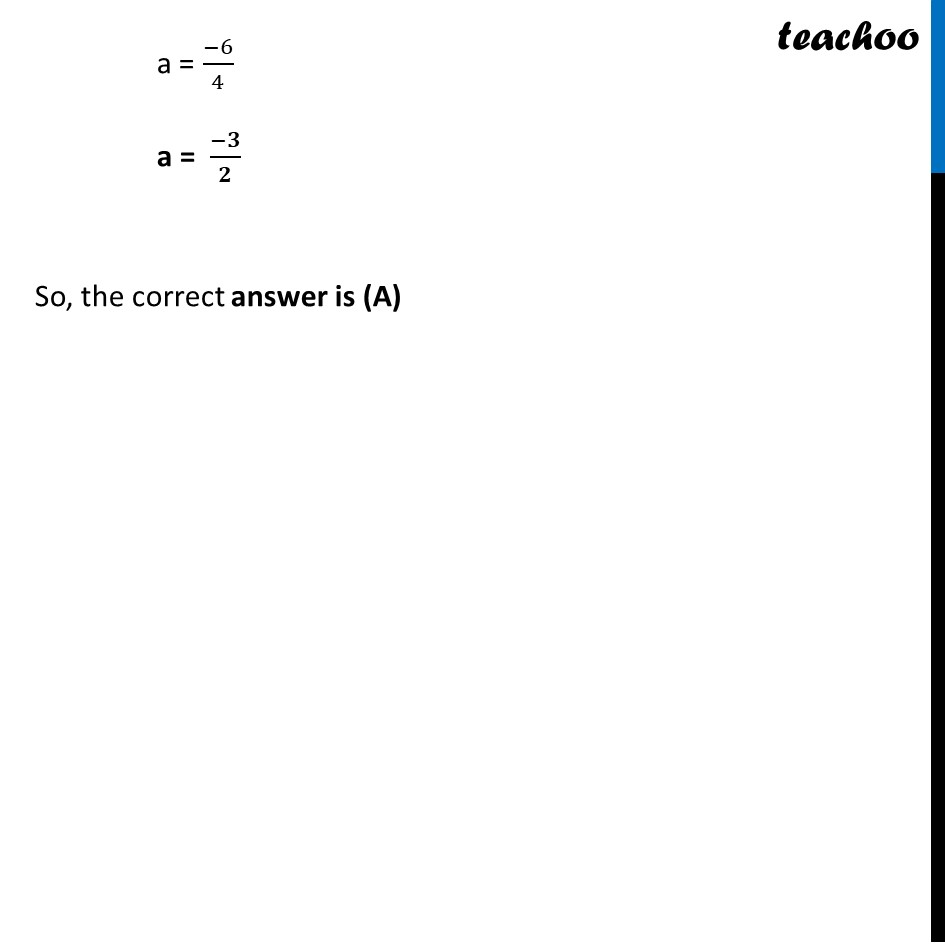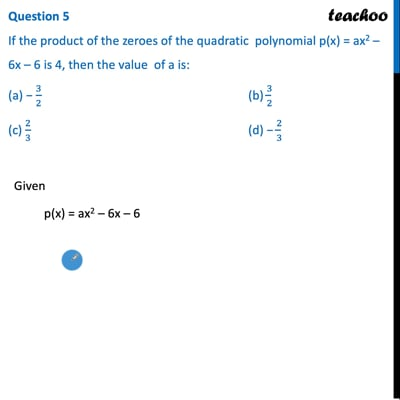This video is only available for Teachoo black users

Introducing your new favourite teacher - Teachoo Black, at only ₹83 per month

### Transcript

Question For a linear polynomial kx + c, k ≠ 0, the graph of y = kx + c is a straight line which intersects the X-axis at exactly one point, namely, ((−𝑐)/𝑘,0), Therefore, the linear polynomial kx + c, k ≠ 0, has exactly one zero, namely, the X-coordinate of the point where the graph of y = kx + c intersects the X-axis. Give answer the following questions: Question 1 If a linear polynomial is 2x + 3, then the zero of 2x + 3 is: (a) 3/2 (b) − 3/2 (c) 2/3 (d) − 2/3 Let p(x) = 2x + 3 Finding zero p(x) = 0 2x + 3 = 0 2x = – 3 x = (−𝟑)/𝟐 So, the correct answer is (B) Question 2 The graph of y = p(x) is given in figure below for some polynomial p(x). The number of zero/zeroes of p(x) is/are: (a) 1 (b) 2 (c) 3 (d) 0 Number of zeroes is equal to number of times parabola intersects the x-axis Since the graph does not intersect the X-axis, ∴ Number of zeroes = 0 So, the correct answer is (d) Question 3 If 𝛼 and 𝛽 are the zeroes of the quadratic polynomial x2 – 5x + k such that 𝛼 – 𝛽 = 1, then the value of k is: (a) 4 (b) 5 (c) 6 (d) 3 Let p(x) = x2 – 5x + k Now, Sum of zeros = 𝒄/𝒂 𝛼 + 𝛽 = (−(−5))/1 𝛼 + 𝛽 = 5 Also given, 𝜶 − 𝜷 = 1 Product of zeros = 𝒄/𝒂 𝛼𝛽 = 𝑘/1 𝛼𝛽 = k Adding (1) and (2) 𝛼 + 𝛽 + 𝛼 − 𝛽 = 5 + 1 2𝛼 = 6 𝛼 = 6/2 𝛼 = 3 Putting 𝛼 = 3 in (1) 𝛼 + 𝛽 = 5 3 + 𝛽 = 5 𝛽 = 5 − 3 𝛽 = 2 Now, from (3) 𝛼𝛽 = k 3 × 2 = k 6 = k k = 6 So, the correct answer is (C) Question 4 If 𝛼 and 𝛽 are the zeroes of the quadratic polynomial p(x) = 4x2 + 5x + 1, then the product of zeroes is: (a) −1 (b) 1/4 (c) −2 (d) − 5/4 Given p(x) = 4x2 + 5x + 1 Now, Product of Zeros = 𝑐/𝑎 = 𝟏/𝟒 So, the correct answer is (B) Question 5 If the product of the zeroes of the quadratic polynomial p(x) = ax2 – 6x – 6 is 4, then the value of a is: (a) − 3/2 (b) 3/2 (c) 2/3 (d) − 2/3 Given p(x) = ax2 – 6x – 6 Here, Product of zeroes = 𝑐/𝑎 4 = (−𝟔)/𝒂 4a = −6 a = (−6)/4 a = (−𝟑)/𝟐 So, the correct answer is (A)